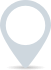# Net profit margin calculator

The net profit margin is a profitability ratio that measures the percentage of revenue that results in net profit.\$
.00
\$
.00

This calculator is intended for illustration purposes only and exact payment terms should be agreed with a lender before taking out a loan.

Net profit margin

-

## How to calculate net profit margin

Net profit margin is calculated using the following formula:

Net Profit Margin = (Net Profit / Total Revenue) * 100

To calculate the net profit margin, follow these steps:

1. Determine your net profit: Calculate your net profit using the formula mentioned earlier, which is the difference between total revenue and total expenses.

2. Calculate your total revenue: This includes all the income generated from sales, services, or any other sources.

3. Apply the formula: Divide your net profit by your total revenue, then multiply the result by 100 to get the net profit margin percentage.

The net profit margin is a useful metric for evaluating the profitability of a business. A higher net profit margin indicates that a higher percentage of revenue is converted into profit, which is generally considered favourable. However, it’s important to consider industry standards and compare the net profit margin with competitors or historical data to gain a better perspective on your business’s performance.

### Clever finance tips and the latest news

#### delivered to your inbox, every week